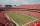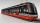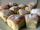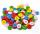# Certificate

There is 31 students in a class. From mathematics was'nt worse mark than 2. Average mark in mathematics was 2.

How many students have mark 1 and how many mark 2?

Result

n(1) =  0
n(2) =  31

#### Solution:(1*a+2*b)/31 = 2
a+b=31

a+2b = 62
a+b = 31

a = 0
b = 31

Calculated by our linear equations calculator.

Leave us a comment of example and its solution (i.e. if it is still somewhat unclear...):

Showing 0 comments:Be the first to comment!#### To solve this example are needed these knowledge from mathematics:

Looking for help with calculating arithmetic mean? Looking for a statistical calculator? Do you have a system of equations and looking for calculator system of linear equations?

## Next similar examples:

1. Hotel roomsIn the 45 rooms, there were 169 guests, some rooms were three-bedrooms and some five-bedrooms. How many rooms were?
2. Classs meanClass size is 30 students, math was not worse than sign 2. Determine the number of pupils who were sign 1 if the class had a 1.4 sign average.
3. TheatroTheatrical performance was attended by 480 spectators. Women were in the audience 40 more than men and children 60 less than half of adult spectators. How many men, women and children attended a theater performance?
4. Three brothersThe three brothers have a total of 42 years. Jan is five years younger than Peter and Peter is 2 years younger than Michael. How many years has each of them?
5. TicketsOn the football tournament ticket cost 45 Kc for standing and 120kč for sitting. Sitting spectators was 1/3 more than standing. The organizers collected a total 12 300 Kc. How many seated and standing seats (spectators)?
6. 5 people5 people have \$122000 and 1 person has \$539000 How much should each person (equally) pay?
7. Two numbersWe have two numbers. Their sum is 140. One-fifth of the first number is equal to half the second number. Determine those unknown numbers.
8. TreesAlong the road were planted 250 trees of two types. Cherry for 60 CZK apiece and apple 50 CZK apiece. The entire plantation cost 12,800 CZK. How many was cherries and apples?
9. Waiting roomIn the waiting room are people and flies. Together they have 15 heads and 50 legs (fly has 6 legs). How many people and flies are in the waiting room?
10. EquationsSolve following system of equations: 6(x+7)+4(y-5)=12 2(x+y)-3(-2x+4y)=-44
11. Equations - simpleSolve system of linear equations: x-2y=6 3x+2y=4
12. AverageThe arithmetic mean of the two numbers is 71.7. One number is 5. Calculate the second number.
13. Linear systemSolve this linear system (two linear equations with two unknowns): x+y =36 19x+22y=720
14. MushroomsEva and Jane collected 114 mushrooms together. Eve found twice as much as Jane. How many mushrooms found each of them?
15. TramIn the three-part tram went 206 passengers in front of others, 226 after others and in the middle half of all. How many passengers went in tram total?
16. DonutsIn the dining room at one table sit Thomas, Filip and Martin. Together they eat 36 donuts. How many donuts eaten everyone, when Filip ate twice as much as Thomas and Martin even half over Filip?
17. ButtonsShirt has 6 buttons, blouse has 4 buttons. All buttons are 176. Shirts and blouses are together 36. How many are the shirts and blouses?